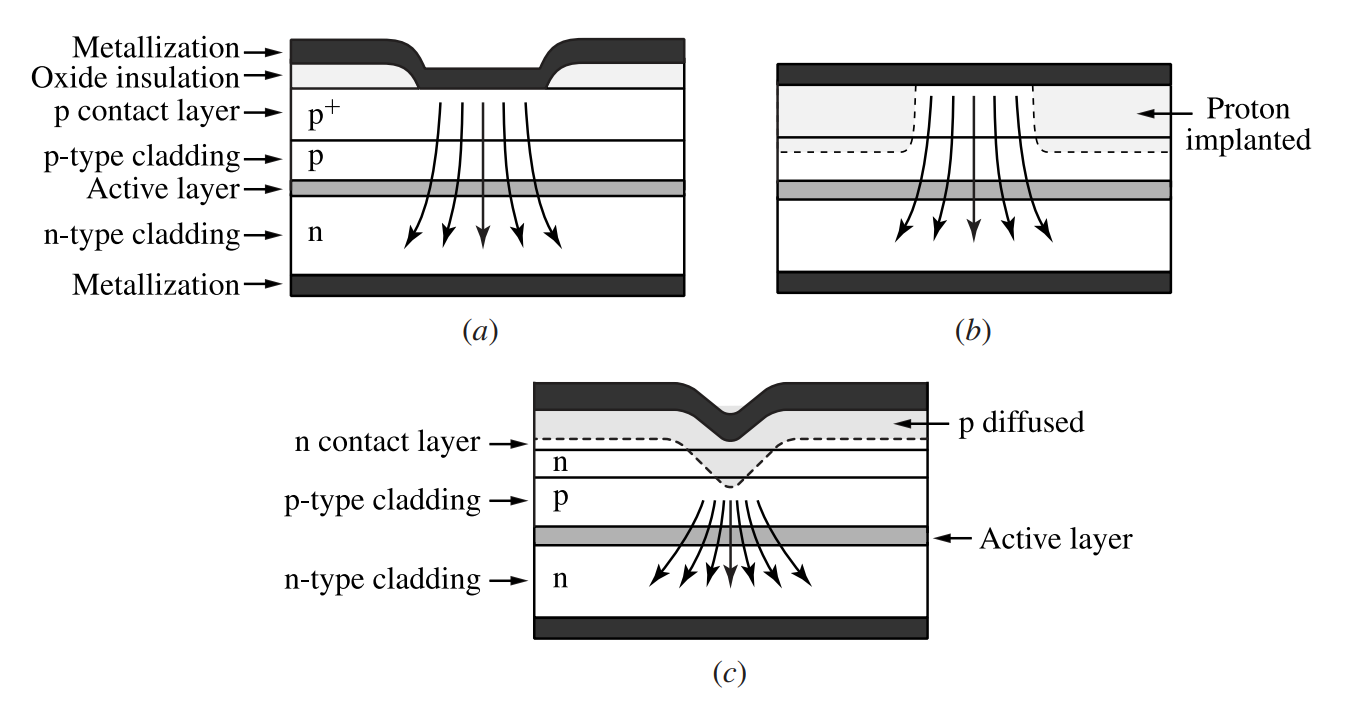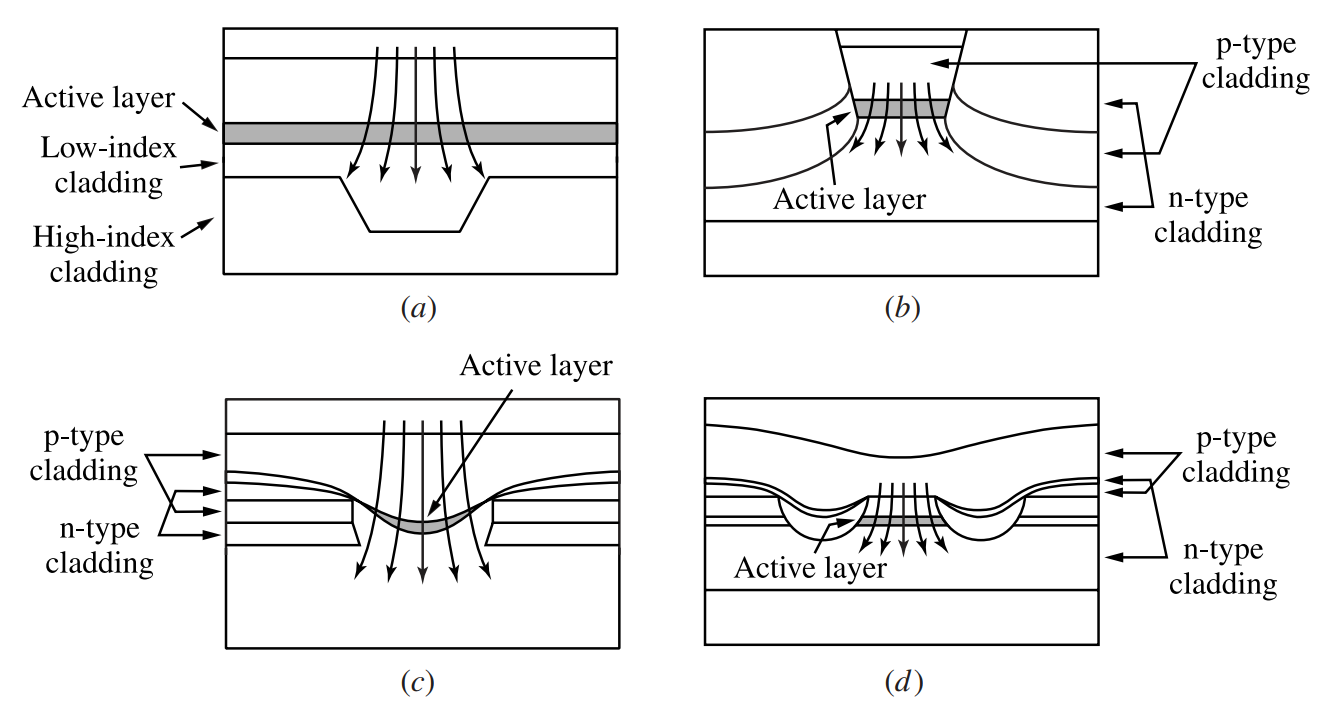# Lateral Structures of Semiconductor Junctions

This is a continuation from the previous tutorial - semiconductor junction structures.

The junction structure of a device determines the carrier and optical field distributions in the vertical direction perpendicular to the junction plane of a device. The carrier and optical field distributions in the transverse directions parallel to the junction plane are determined by the lateral structure.

The lateral structure of a surface-emitting device can have either a broad active area, formed with little or no lateral restrictions on the injected current, or a small active area, formed by restricting the current flow into a confined area, as shown in Figures 13-15(a) and (b), respectively.

Most surface-emitting LEDs are broad-area devices. Some surface-emitting LEDs for fiber-optic applications and the vertical cavity surface-emitting lasers are small-area devices.

For a small-area surface-emitting device, an optical waveguiding structure with index steps in lateral directions can be incorporated in conjunction with the small light-emitting active region for lateral optical confinement. The geometry of this structure is normally symmetric in transverse dimensions.

For a laser, a small waveguiding area is important for single-transverse-mode emission. As a result, the emission of the device forms a circular beam of symmetric divergence with a divergence angle of $$\Delta\theta$$ shown in Figure 13-15(b).

The divergence angle of a device varies significantly among devices of different structural dimensions and emission wavelengths; it also varies under different operating conditions for a given device.

Representative values are $$\Delta\theta=100^\circ$$ for incoherent emission of a surface-emitting LED and $$\Delta\theta=10^\circ$$ for coherent emission of a surface-emitting laser.

The lateral structures of edge-emitting devices have two basic types of geometry: broad-area geometry and stripe geometry, shown in Figures 13-16(a) and (b), respectively.

A broad-area device has no particular structures for restricting current flow to a particular region or for guiding the optical wave in lateral directions parallel to the junction plane.

As a result, the broad-area geometry provides neither lateral carrier confinement nor lateral optical waveguiding.

These deficiencies of broad-area geometry in lateral direction are similar to those of the homojunction in the vertical direction. Such a geometry leads to multiple transverse modes and filaments in the emission profile of the device.

For this reason, almost all practical edge-emitting devices have stripe geometry.

Except for some high-power lasers of special interest, all practical edge-emitting semiconductor lasers have narrow stripes to ensure single-transverse-mode emission. In contrast, edge-emitting LEDs typically have broader stripes than lasers.

There are two types of structures for the stripe geometry: gain-guiding structure and index-guiding structure. Both types of stripe geometry provide lateral confinement for the carriers and the optical field.

Because of the asymmetry between vertical and lateral waveguiding structures, the emission profile of a stripe-geometry device is asymmetric with different vertical and lateral divergence angles: $$\Delta\theta_{\perp}$$ and $$\Delta\theta_{\parallel}$$, respectively, as shown in Figure 13-16(b).

Because the width $$w$$ of the stripe is normally much larger than the thickness $$d$$ of the active layer, $$\Delta\theta_{\perp}\gt\Delta\theta_{\parallel}$$ for the coherent emission of a laser but $$\Delta\theta_{\perp}\lt\Delta\theta_{\parallel}$$ for the incoherent emission of an LED.

Both vertical and lateral divergence angles vary significantly among devices of different structural dimensions and emission wavelengths, as well as under different operating conditions for a given device.

Representative values are $$\Delta\theta_{\perp}=30^\circ$$ and $$\Delta\theta_{\parallel}=120^\circ$$ for the incoherent emission of an edge-emitting LED and $$\Delta\theta_{\perp}=30^\circ$$ and $$\Delta\theta_{\parallel}=10^\circ$$ for the coherent emission of a single-transverse-mode edge-emitting laser.

## Gain-Guiding Stripe Geometry

The gain-guiding stripe geometry in a again-guided device is formed by injecting the current within a narrow stripe, which typically has a width ranging from a few micrometers for a gain-guided laser to a few tens of micrometers for an LED.

No additional lateral structure is incorporated in the device. The current stripe defines the longitudinal direction of the edge-emitting device.

Figure 13-17 shows the basic characteristics of a gain-guided device. There are a few different structures, shown in Figure 13-18, that can be used to create the gain-guiding stripe.Figure 13-17.  (a) Basic structure, (b) excess carrier distribution, (c) refractive index profile, and (d) lateral optical field distribution of a gain-guided stripe-geometry device.Figure 13-18.  Structures of gain-guided devices: (a) oxide stripe, (b) proton-bombarded stripe, and (c) V-groove stripe.

As is shown in Figure 13-17(b), a stripe of concentrated carriers in the active layer is formed along the longitudinal direction of a gain-guided device.

In a semiconductor, an increase in carrier concentration is generally accompanied by a decrease in refractive index. This phenomenon is known as the antiguidance effect. In a semiconductor gain medium, it is often described by an experimentally measurable antiguidance factor, also known as the linewidth enhancement factor, defined as

$\tag{13-61}b=\frac{\partial{n}'/\partial{N}}{\partial{n}^"/\partial{N}}=-\frac{2\omega}{c}\frac{\partial{n}'/\partial{N}}{\partial{g}/\partial{N}}=-\frac{4\pi}{\lambda}\frac{\partial{n}'/\partial{N}}{\partial{g}/\partial{N}}$

where $$n'$$ and $$n^"$$ are the real and imaginary parts of the refractive index and $$g$$ is the optical gain coefficient.

The antiguidance factor is a function of material, optical wavelength, temperature, and other operating conditions. It typically ranges between 2 and 6 for most semiconductor structures, but it can be as small as 1 and as large as 10.

The antiguidance effect results in an antiguiding index profile, shown in Figure 13-17(c), that tends to spread the optical field laterally instead of confining it. This effect is not important for an LED, which does not require an optical gain and has incoherent emission.

For a semiconductor laser, this effect is counteracted by the optical gain that is concentrated along the stripe. Stimulated amplification of the coherent laser field by this stripe of optical gain results in a gain-guiding effect for a laser beam to propagate along the longitudinal direction of the stripe.

Nevertheless, the antiguiding index profile of a gain-guided laser still causes the beam to expand laterally to a certain degree.

The stripe width of a gain-guiding device varies between 10 and 200 μm, depending on intended applications. A wide stripe allows a large current to be injected for a high-power device, but it also makes the threshold current high in the case of a laser.

A gain-guided laser tends to oscillate in multiple transverse modes, making it difficult to focus or collimate. Gain-guided LEDs are common because they are not subject to these limitations.

Gain-guided lasers find important applications in the areas where high power and high conversion efficiency are needed but coherence and collimation of the beam are not very important, such as in pumping solid-state lasers or fiber lasers.

Example 13-8

Use the results obtained in Example 13-4 [refer to the optical gain in semiconductors tutorial] to estimate the carrier-induced index change in GaAs at 850 nm for an injected carrier density of $$N=2.83\times10^{24}\text{ m}^{-3}$$ if the antiguidance factor is $$b=3$$.

From Example 13-4(c) [refer to the optical gain in semiconductors tutorial], we find that

$\frac{\partial{g}}{\partial{N}}=\sigma=3.37\times10^{-20}\text{ m}^2$

for GaAs at 850nm at $$300\text{ K}$$. To estimate the index change, we assume that the refractive index changes linearly with carrier density. Thus, by using (13-61), we have

$\frac{\Delta{n}}{\Delta{N}}\approx\frac{\partial{n'}}{\partial{N}}=-\frac{b\lambda}{4\pi}\frac{\partial{g}}{\partial{N}}=-\frac{b\lambda\sigma}{4\pi}$

For $$\Delta{N}=N=2.83\times10^{24}\text{ m}^{-3}$$, we have

$\Delta{n}\approx-\frac{b\lambda\sigma{N}}{4\pi}=-\frac{3\times850\times10^{-9}\times3.37\times10^{-20}\times2.83\times10^{24}}{4\pi}=-1.94\times10^{-2}$

Compared to the base refractive index of $$n=3.65$$ at 850 nm for GaAs in the absence of injected carriers, this carrier-induced index change is small. Nevertheless, such a small index reduction can cause a significant antiguiding effect to spread the distribution of an optical field if the optical field is not otherwise confined by a waveguiding index profile.

## Index-Guiding Stripe Geometry

For truly effective lateral optical confinement, an index-guiding structure has to be used. In an index-guiding structure, the lateral waveguide is formed by introducing a lateral index profile around the active region along the stripe where current is injected.

As a result, both carrier confinement and optical waveguiding in the lateral direction are accomplished in a manner similar to the way a DH provides confinement for both carriers and optical field in the vertical direction.

The basic characteristics of an index-guided device are illustrated in Figure 13-19. There are many different index-guiding structures. A few examples are shown in Figure 13-20.Figure 13-19.  (a) Basic structure, (b) excess carrier distribution, (c) refractive index profile, and (d) lateral optical field distribution of an index-guided stripe-geometry device.Figure 13-20.  Structures of index-guided devices: (a) channeled-substrate planar (CSP) structure, (b) planar buried heterostructure (PBH), (c) buried crescent heterostructure, and (d) double-channel planar buried heterostructure (DC-PBH). Note that current flow is defined by the contact geometry, which is not shown in these illustrations.

In an index-guiding structure, the antiguidance effect is not important because the physical index steps that create the lateral waveguide are larger than the small changes in the refractive index caused by the injected carriers in the active region.

Consequently, as the operating parameters, such as the injection current or the operating temperature, are varied, the output beam characteristics, including its profile, size, and divergence, of an index-guided device are more stable than those of a gain-guided device.

In addition, because the bandgap steps associated with the physical index steps create energy barriers for carriers like those in a DH, a high concentration of carriers can be injected and confined within the active region of an index-guiding device.

Whereas the lateral carrier distribution in a gain-guided device is determined by the lateral spread of the current injected into the device and the lateral diffusion of carriers in the active layer, the lateral carrier distribution in an index-guided device is defined by the width of the index-guiding stripe.

Therefore, it is possible to increase the carrier concentration by using a narrow index-guiding stripe to increase the efficiency of an LED and to lower the threshold of a laser. For single-transverse-mode lasers, the width of the stripe can be as narrow as 1-2 μm.

Example 13-9

An edge-emitting GaAs laser with an index-guiding stripe geometry emits at 850 nm wavelength. The vertical and lateral far-field divergence angles of the laser beam are $$\Delta\theta_{\perp}=30^\circ$$ and $$\Delta\theta_{\parallel}=10^\circ$$, respectively. What are the vertical and lateral sizes of the beam at the output facet of the laser?

Because $$\Delta\theta_{\perp}\ne\Delta\theta_{\parallel}$$, the laser spot is elliptical with $$w_{\perp}\ne{w}_{\parallel}$$. Because the divergence angles are measured in free space, we take the refractive index of $$n=1$$ for free space to find the spot size at the beam waist, which is located at the output facet of the laser.

We have $$\Delta\theta_{\perp}=30^\circ=\pi/6\text{ rad}$$ and $$\Delta\theta_{\parallel}=10^\circ=\pi/18\text{ rad}$$. Thus, by using (137) [refer to the Gaussian beam tutorial] for Gaussian beam divergence, we find that

$w_{0\perp}=\frac{2\lambda}{\pi\Delta\theta_{\perp}}=\frac{2\times850\times10^{-9}}{\pi\times\pi/6}\text{ m}=1.03\text{ μm}$

and

$w_{0\parallel}=\frac{2\lambda}{\pi\Delta\theta_{\parallel}}=\frac{2\times850\times10^{-9}}{\pi\times\pi/18}\text{ m}=3.1\text{ μm}$

The near-field spot at the laser facet is elliptical with $$w_{0\parallel}=3w_{0\perp}$$ because $$\Delta\theta_{\perp}=3\Delta\theta_{\parallel}$$.

Such small beam sizes in both vertical and lateral directions can be made possible only by a laser structure with index guiding in both vertical and lateral dimensions.

The next tutorial covers the topic of light-emitting diodes (LEDs)